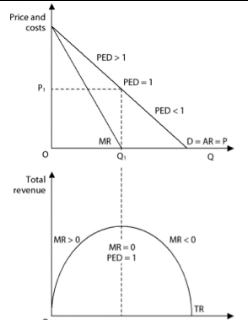## Monday, November 7, 2016

### 2008 B Micro FRQ #1

2008 B Micro FRQ #1

(A) Suppose the firm produces at the profit-max output (quantity). Using the labelling on the graph, identify each of the following.

(i) Level of output. Explain.

First, you find the profit max (MC = MR) and then draw a line straight down to the quantity. Profit is maximised at MC = MR so this is where the company should produce to maximise profit.

(ii) Price

(B) Suppose the firm maximises at the max revenue output. Using the labelling on the graph identify each of the following.

(i) Level of output. Explain.

Understand that max revenue is where the marginal revenue curve equals zero. Why? At that quantity of good produced (Q3) there is still some profit being made. Production at (Q4) would cause the company to incur a loss.

Why would a company choose to maximise revenue instead of maximising profit? Well, perhaps you are a new business that needs to build up a clientele. So, you can sell at a cheaper price and get some market share.

(ii) PriceTotal revenue for a monopoly is maxed where MR = 0, to produce more quantity would produce a loss on each unit produced

(C) Suppose the government regulates the firm's price to produce the allocatively efficient level of output (quantity).

(i) The price the government would require the firm to set. Explain.Know this!!!!!
The government would require the firm to set the price where price (P) is equal to the (MC) or at the socially allocatively efficient level. This can be labelled (P = MC) or sometimes where (D = MC) same/same.

(ii) Alocatively efficient level of output (quantity).

(D) Suppose the firm is producing at the allocatively efficient level of output.

(i) Would it be earning a profit or incurring a loss? Explain.

A loss as at the quantity of Q4 and a price of P1, the ATC is higher than the revenues generated.

(ii) Identify the area of loss or profit at the allocatively efficient level of output.

(E) Identify the Consumer surplus at the allocatively efficient level of production.

(F) Suppose the government allows the firm to set a price that just covers its opportunity costs. Identify the price the regulators would allow.

The Regulators would allow the firm to choose the fair return/ break-even price so as to cover its expensive,, to choose a lower price would require a subsidy from the government to keep the firm in business.

Fair-Return/ Break-Even price =  where, P = ATC

Notice that the Q3 price is lower with regulation than with a profit maximising or revenue maximising monopoly and there is more quantity produced, closer to the socially optimal quantity. The regulated monopoly will produce more quantity at a lower price.